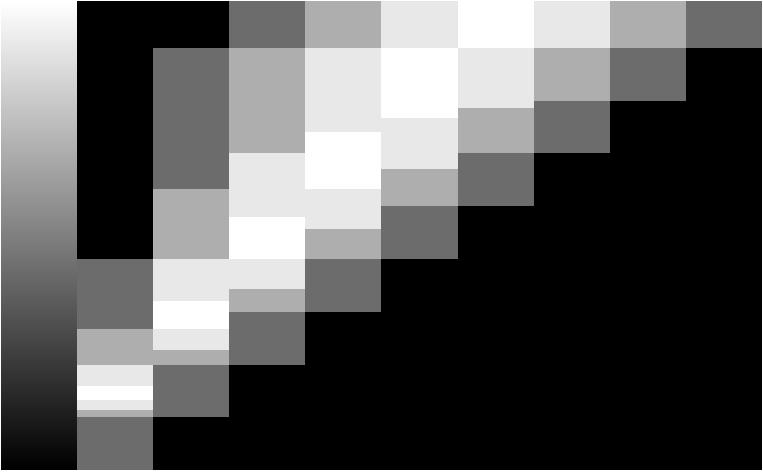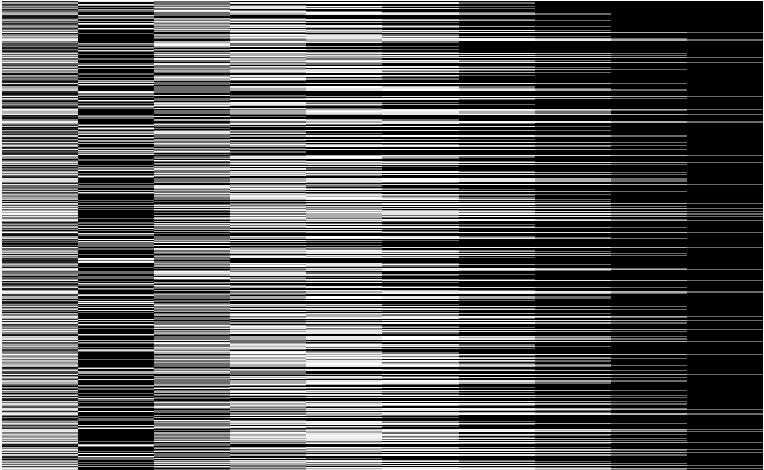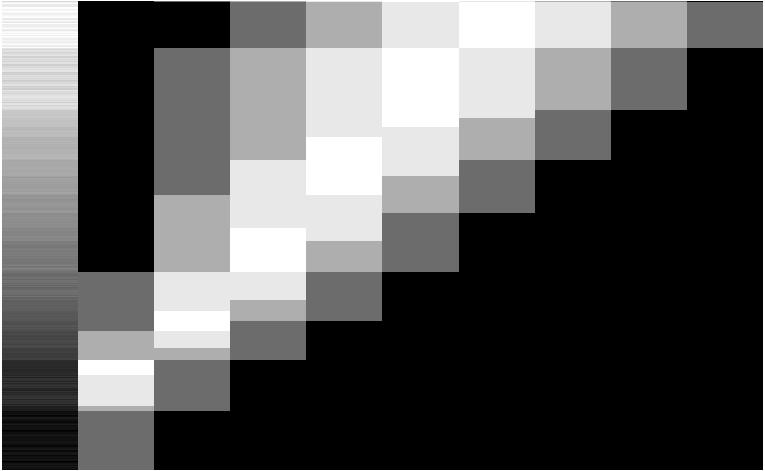Example 15.  Similarity (SSD) Sorting of Signals Displayed in Contour Plots

For a signal of length (c) that has only two possible states (0 and 1, i.e., binary), there are 2c possible signals that can be transmitted.  If this information is contained in a number (n) of signals of length c, there are 2n*c possibilities.  If the number of states is increased to 10 levels, then there are 10n*c possibilities.  The latter grouping of signals could look like a matrix of c columns and n rows with the numbers 1-10 as elements.

In the first part of this example, we will create a mock data set with 800 signals than have 10 channels each with 10 levels per channel.  If one thinks of this as 10 grayscale possibilities per each element of an (n = height and c = width) image, that yields 10800*10 = 108000 possible 'images'.

We shall make only one such image, and then: 1) display it, 2) randomize the rows, 3) display the randomized image, 4) sort the randomized image by rows using a similarity criterion, and 5) recompose the original image from the scrambled image.  Obviously, as the number of rows, columns, and grayscale values increase, the sorting algorithm's efficiency becomes very important for time of computation.  We will use a sum-of-the-squares-of-the-difference (SSD) criterion to group rows that have the least difference.  The control structure and the manner in which the SSD algorithm is applied must also be efficient:  One does not want to resort rows that have already been sorted.

To create mock signal data in a 800 x 10 format with 10 levels per channel, we have used simple control structure (For, and Floor functions) to make a nested set of signals.  This procedure creates an image where it appears that the maximum signal strength migrates from right to left as one descends from top to bottom in the resulting image (see Image 15.1).Image 15.1.  Mock data set generated with 800 rows, 10 columns, and 10 levels per element.  Channel 1 is a grayscale row marker, where the value of gray corresponds to signal number, from 1 - 100, normalized such that 1 is black and 100 is white.

In the next step, we scramble this image by building a new matrix, where a random number generator is called to select rows from the original matrix to be inserted into the new (scrambled) matrix.  A given row in the original matrix (from Image 15.1) can be selected more than once or not at all.  Given the redundancy of the original matrix (due to flooring of certain values), the scrambled matrix has a good sampling of the diversity present in the original image.  The row-scrambled image is shown in 15.2 .Image 15.2. This image consists of rows randomly selected from 15.1. Note that the grayscale in channel 1 (that denotes row number) is very scrambled.

Now our job is to recreate the original image as best we can.  Thinking about this in terms of individual rows, we have 800 possibilities to pick from for the first row, 799 possibilities for the second row, ... and only one possibility for the last row.  Thus there are n! (i.e., 800!) possible ways to rebuild the image by row.  The problem is actually not this bad, because the original data set had left-to-right and top-to-bottom structuring of grayscale levels, as described above.  Furthermore, if we tell the sorting algorithm which row is number one, then the problem becomes one of selecting the next (most similar) row and inserting it as row 2.  Having done that, row 2 then becomes the 'target' and its most similar row is then found - with the exception of rows that have already been sorted and placed.  And, etc., for the entire matrix sorting.  By the time we have sorted 90% the image, there are only 80 rows left that are not already picked.  The final row is the one remaining unsorted row, and hence there are no combinatorial possibilities.

In this sorting scenario, we have reduced n! to a sum of all the numbers between 1 and n.  A well known student once told his teacher how to sum this:  Split the summation into two parts, numbers (1-400) and (401-800); reverse the order of the second list (1-400) and (800-401).  Add the list by positional elements.  All sums are 801 and there are 400 of them.  The answer to the summation question is:  400 x 801 = 320,400.  Using a similarity sort, we have reduced this image re-building problem from 800! to 320,400.  It is an entirely different issue to code this efficiently.

Our only other problem is to use a robust comparison criterion when comparing rows for similarity.  As mentioned above, we shall use SSD over all nine of the columns that contain data.  Recall that the first column is a grayscale value encoding row number.  It is thoroughly scrambled in Image 15.2.  Our success or failure will be realized quickly by examining whether the grayscale column in 15.1 is recreated after sorting, as shown in 15.3.Image 15.3.  Sorting of Image 15.2 by row using an SSD similarity criterion.  Column one was not included in the sorting algorithm, and its continuity shows that the sort is successful.  Many of the signals are redundant, thus their grayscale indicator is somewhat scrambled within each grouping of similar and/or identical signals.

One of the most striking features of Mathematica that people first saw 2 decades ago was its ability to plot data.  A single plotting command graphs (Image 15.4) the data that was shown in Image 15.3 .Image 15.4.  Mathematica graphing of the Image 15.3 data.  Notice the quasi-continuity of the grayscale ramp in column one as an indicator of a successful sort.

This example is designed to show 'similarity sorted contour plots', a term coined by my then graduate student, Adam Arkin, and myself in ca. 1990 at MIT.  Adam is now a professor at UC Berkeley.  This sorting and display technology has been extensively utilized and commercialized by Kairos Scientific Inc., under the stewardship of my former postdoc and partner, Dr. Mary M. Yang.  Dr. Yang is currently the CEO and President of Kairos.  Similarity sorts are a critical component of Kairos' digital imaging spectrophotometers (DIS) illustrated at http://www.et-al.com/pdf/SinglePixel1.pdf .  A USA patent for using DIS in enzymology can be found at www.uspto.gov by searching the patent database for patent number 5,914,245 .

Suggestions for further work, include:  automatically identifying and counting different signal groups (~20 in this example), improving the sorting algorithm (see 'heap sort' and others on the web), and working with real data that has noise (e.g., hyperspectral remote sensing data or temporal data).  For this example, the Mathematica code runs in a ~ 30 seconds, thus indicating that much larger and complex data sets can be analyzed.  Mathematica also provides for compiling parts of the code that are computation intensive.  Implementing such compilation of the sorting control flows and SSD algorithm is another suggestion for further work.  In addition, as the number of levels per channel increases, pseudocolor (rather than grayscale) is useful for highlighting subtle differences among the signals.

Code in .nb and .PDF format.